# CBSE Question Paper 2017 Class 12 Physics## myCBSEguide App

CBSE, NCERT, JEE Main, NEET-UG, NDA, Exam Papers, Question Bank, NCERT Solutions, Exemplars, Revision Notes, Free Videos, MCQ Tests & more.

CBSE Question Paper 2017 class 12 Physics conducted by Central Board of Secondary Education, New Delhi in the month of March 2017. CBSE previous year question papers with solution are available in myCBSEguide mobile app and cbse guide website. The Best CBSE App for students and teachers is myCBSEguide which provides complete study material and practice papers to cbse schools in India and abroad.

Question Paper 2017 class 12 Physics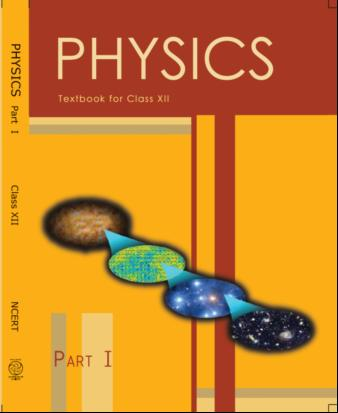## Class 12 Physics chapters wise list

1. Electric Charges and Fields
2. Electrostatic Potential and Capacitance
3. Current Electricity
4. Moving Charges and Magnetism
5. Magnetism and Matter
6. Electromagnetic Induction
7. Alternating Current
8. Electromagnetic Waves
9. Ray Optics and Optical Instruments
10. Wave Optics
11. Dual Nature of Radiation and Matter
12. Atoms
13. Nuclei
14. Semiconductor Electronic: Material, Devices and Simple Circuits
15. Communication Systems

## CBSE Question Paper 2017 Class 12 Physics

### General Instructions:

1. All questions are compulsory. There are 26 questions in all.
2. This question paper has five sections: Section A, Section B, Section C, Section D and Section E.
3. Section A contains five questions of one mark each, Section B contains five questions of two marks each, Section C contains twelve questions of three marks each, Section D contains one value based question of four marks and Section E contains three questions of five marks each.
4. There is no overall choice. However, an internal choice has been provided in one question of two marks, one question of three marks and all the three questions of five marks weightage. You have to attempt only one of the choices in such questions.
5. You may use the following values of physical constants wherever necessary :

c = 3 × 108m/s, h = 6.63 × 10–34 Js
e = 1.6 × 10–19 C,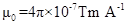= 8.854 × 10–12 C2 N–1 m–2,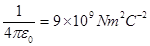Mass of electron = 9.1 × 10–31 kg, Mass of neutron = 1.675 × 10–27 kg
Mass of proton = 1.673 × 10–27 kg, Avogadro’s number = 6.023 × 1023 per gram mole
Boltzmann constant = 1.38 × 10–23 JK–1

### SECTION – A

1. A point charge Q is placed at point ‘O’ as shown in the figure. Is the potential at point A, i.e. VA , greater, smaller or equal to potential, VB , at point B, when Q is (i) positive, and (ii) negative charge ? (1)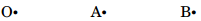2. Write the expression for speed of electromagnetic waves in a medium of electrical permittivity ε and magnetic permeability μ. (1)
3. Does the magnifying power of a microscope depend on the colour of the light used ? Justify your answer. (1)
4. Draw logic symbol of an OR gate and write its truth table. (1)
5. A photosensitive surface emits photoelectrons when red light falls on it. Will the surface emit photoelectrons when blue light is incident on it ? Give reason. (1)

### SECTION B

6. Find the intensity at a point on a screen in Young’s double slit experiment where the interfering waves of equal intensity have a path difference of (i) λ/4, and (ii) λ/3. (2)
7. Write two points of difference between intrinsic and extrinsic semiconductors. (2)
8. Distinguish between broadcast mode and point-to-point mode of communication and give one example for each. (2)

9. A light bulb and a solenoid are connected in series across an ac source of voltage. Explain, how the glow of the light bulb will be affected when an iron rod is inserted in the solenoid. (2)
10. Use the mirror equation to show that an object placed between f and 2f of a concave mirror forms an image beyond 2f. (2)
OR (a) State the condition under which a large magnification can be achieved in an astronomical telescope.
(b) Give two reasons to explain why a reflecting telescope is preferred over a refracting telescope.

### SECTION C

11. (a) Define the term ‘modulation index,’ used in communication system. Why is its value kept less than or equal to one?

(b) A message signal of frequency 10 kHz and peak voltage of 10 V is used to modulate a carrier frequency 1 MHz and peak voltage 10 V. Determine the (i) modulation index, and (ii) side bands produced. (3)
12. Using Bohr’s postulates, derive the expression for the orbital period of the electron moving in the nth orbit of hydrogen atom. (3)

13. A charge Q is distributed uniformly over a metallic sphere of radius R. Obtain the expressions for the electric field (E) and electric potential (V) at a point 0 < x < R. Show on a plot the variation of E and V with x for 0 < x < 2R. (3)
14. In the given circuit, with steady current, calculate the potential difference across the capacitor and the charge stored in it. (3)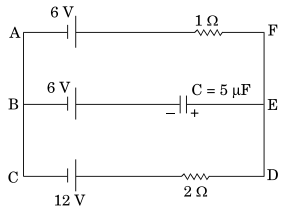15. A long charged cylinder of linear charge density + λ1 is surrounded by a hollow coaxial conducting cylinder of linear charge density -λ2. Use Gauss’s law to obtain expressions for the electric field at a point (i) in the space between the cylinders, and (ii) outside the larger cylinder.
16. Using Biot-Savart law, deduce the expression for the magnetic field at a point (x) on the axis of a circular current carrying loop of radius R. How is the direction of the magnetic field determined at this point ? (3)

OR The figure shows three infinitely long straight parallel current carrying conductors. Find the
(i) magnitude and direction of the net magnetic field at point A lying on conductor 1,
(ii) magnetic force on conductor 2.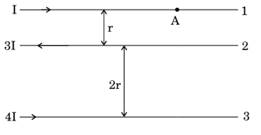17. (a) State the law of radioactive decay. Write the SI unit of ‘activity’.
(b) There are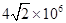radioactive nuclei in a given radioactive sample. If the half-life of the sample is 20 s, how many nuclei will decay in 10 s ? (3)

18. (a) How are electromagnetic waves produced ? Explain.
(b) A plane electromagnetic wave is travelling through a medium along the +ve z-direction. Depict the electromagnetic wave showing the directions of the oscillating electric and magnetic fields. (3)

19. A source of ac voltage v = v0 sin ωt, is connected across a pure inductor of inductance L. Derive the expressions for the instantaneous current in the circuit. Show that average power dissipated in the circuit is zero. (3)

20. (a) Draw a plot showing the variation of photoelectric current with collector potential for different frequencies but same intensity of incident radiation.
(b) Use Einstein’s photoelectric equation to explain the observations from this graph.
(c) What change will you observe if intensity of incident radiation is changed but the frequency remains the same? (3)

21. (a) State the condition under which a charged particle moving with velocity v goes undeflected in a magnetic field B.
(b) An electron, after being accelerated through a potential difference of 104 V, enters a uniform magnetic field of 0·04 T, perpendicular to its direction of motion. Calculate the radius of curvature of its trajectory. (3)

22. A monochromatic light of wavelength λ is incident normally on a narrow slit of width ‘a’ to produce a diffraction pattern on the screen placed at a distance D from the slit. With the help of a relevant diagram, deduce the conditions for obtaining maxima and minima on the screen. Use these conditions to show that angular width of central maximum is twice the angular width of secondary maximum. (3)

### SECTION D

23. Sunil and his parents were travelling to their village in their car. On the way his mother noticed some grey coloured panels installed on the roof of a low building. She enquired from Sunil what those panels were and Sunil told his mother that those were solar panels.
(a) What were the values displayed by Sunil and his mother ? State one value for each.
(b) In what way would the use of solar panels prove to be very useful?
(c) Name the semiconductor device used in solar panels. Briefly explain with the help of a diagram, how this device works. (4)

### SECTION E

24. (a) (i) State the principle on which a potentiometer works. How can a given potentiometer be made more sensitive ?
(ii) In the graph shown below for two potentiometers, state with reason which of the two potentiometers, A or B, is more sensitive.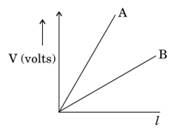(b) Two metallic wires, P1 and P2 of the same material and same length but different cross-sectional areas, A1 and A2 are joined together and connected to a source of emf. Find the ratio of the drift velocities of free electrons in the two wires when they are connected (i) in series, and (ii) in parallel.

OR (a) Define the capacitance of a capacitor. Obtain the expression for the capacitance of a parallel plate capacitor in vacuum in terms of plate area A and separation d between the plates.

(b) A slab of material of dielectric constant K has the same area as the plates of a parallel plate capacitor but has a thickness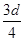. Find the ratio of the capacitance with dielectric inside it to its capacitance without the dielectric.

25. (a) State Faraday’s law of electromagnetic induction.

(b) The magnetic field through a circular loop of wire 12 cm in radius and 8·5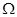resistance, changes with time as shown in the figure. The magnetic field is perpendicular to the plane of the loop. Calculate the induced current in the loop and plot it as a function of time.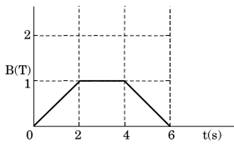(c) Show that Lenz’s law is a consequence of conservation of energy.

OR (a) Describe, with the help of a suitable diagram, the working principle of a step-up transformer. Obtain the relation between input and output voltages in terms of the number of turns of primary and secondary windings and the currents in the input and output circuits.

(b) Given the input current 15 A and the input voltage of 100 V for a step-up transformer having 90% efficiency, find the output power and the voltage in the secondary if the output current is 3 A.

26. (a) A point object is placed on the principal axis of a convex spherical surface of radius of curvature R, which separates the two media of refractive indices n1 and n2 (n2 > n1). Draw the ray diagram and deduce the relation between the object distance (u), image distance

(v) and the radius of curvature (R) for refraction to take place at the convex spherical surface from rarer to denser medium.

(b) A converging lens has a focal length of 20 cm in air. It is made of a material of refractive index 1·6. If it is immersed in a liquid of refractive index 1·3, find its new focal length.OR (a) Draw the ray diagram showing refraction of light through a glass prism and hence obtain the relation between the refractive index μ of the prism, angle of prism and angle of minimum deviation.

(b) Determine the value of the angle of incidence for a ray of light travelling from a medium of refractive index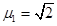into the medium of refractive index μ2 = 1, so that it just grazes along the surface of separation.

These are questions only. To view and download complete question paper with solution install myCBSEguide App from google play store or login to our student dashboard.

## Last Year Question Paper Class 12  Physics 2017

Download class 12 Physics question paper with solution from best CBSE App the myCBSEguide. CBSE class 12 Physics question paper 2017 in PDF format with solution will help you to understand the latest question paper pattern and marking scheme of the CBSE board examination. You will get to know the difficulty level of the question paper.

## Previous Year Question Paper for class 12 in PDF

CBSE question papers 2018, 2017, 2016, 2015, 2014, 2013, 2012, 2011, 2010, 209, 2008, 2007, 2006, 2005 and so on for all the subjects are available under this download link. Practicing real question paper certainly helps students to get confidence and improve performance in weak areas.

To download CBSE Question Paper class 12 Accountancy, Chemistry, Physics, History, Political Science, Economics, Geography, Computer Science, Home Science, Accountancy, Business Studies and Home Science; do check myCBSEguide app or website. myCBSEguide provides sample papers with solution, test papers for chapter-wise practice, NCERT solutions, NCERT Exemplar solutions, quick revision notes for ready reference, CBSE guess papers and CBSE important question papers. Sample Paper all are made available through the best app for CBSE students and myCBSEguide website.